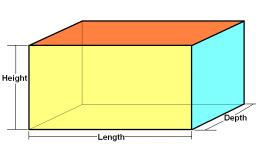# Calculate 2946

The surface of the block is 94 cm2. The lengths of its two edges are a = 3 cm, b = 5 cm. Calculate the length of its third edge. Let's say: From the formula for the block surface, first calculate c.

c =  4 m

### Step-by-step explanation:Did you find an error or inaccuracy? Feel free to write us. Thank you!